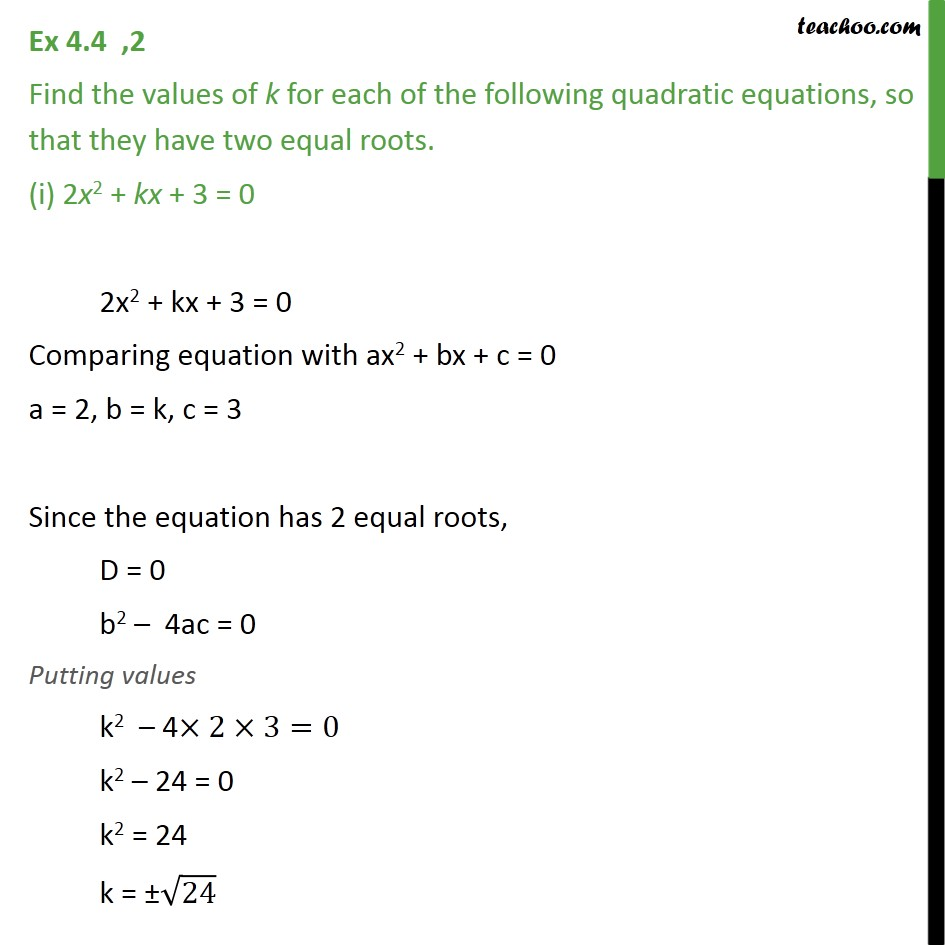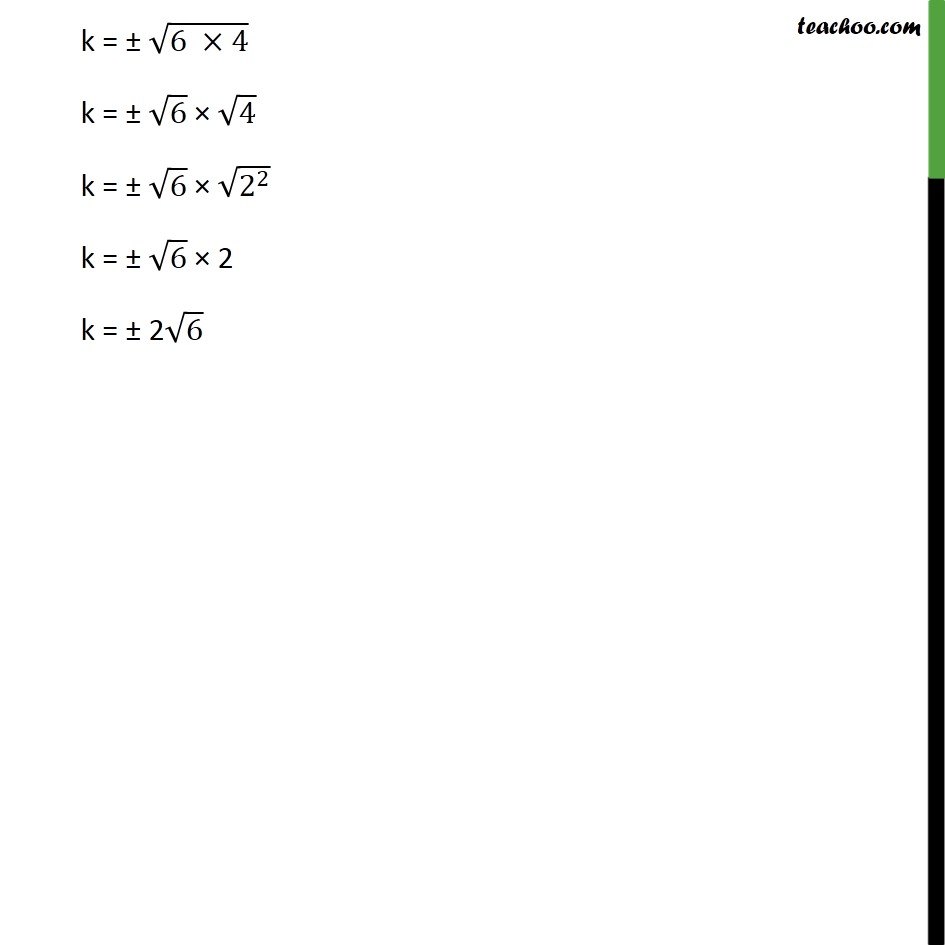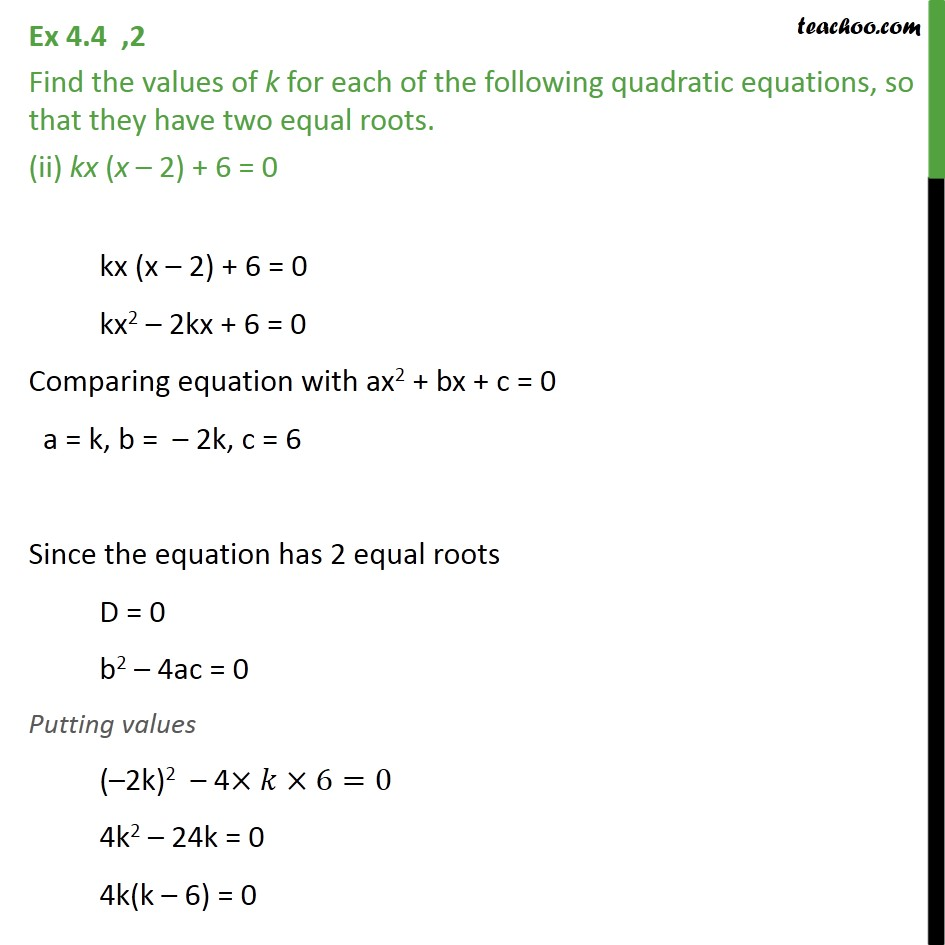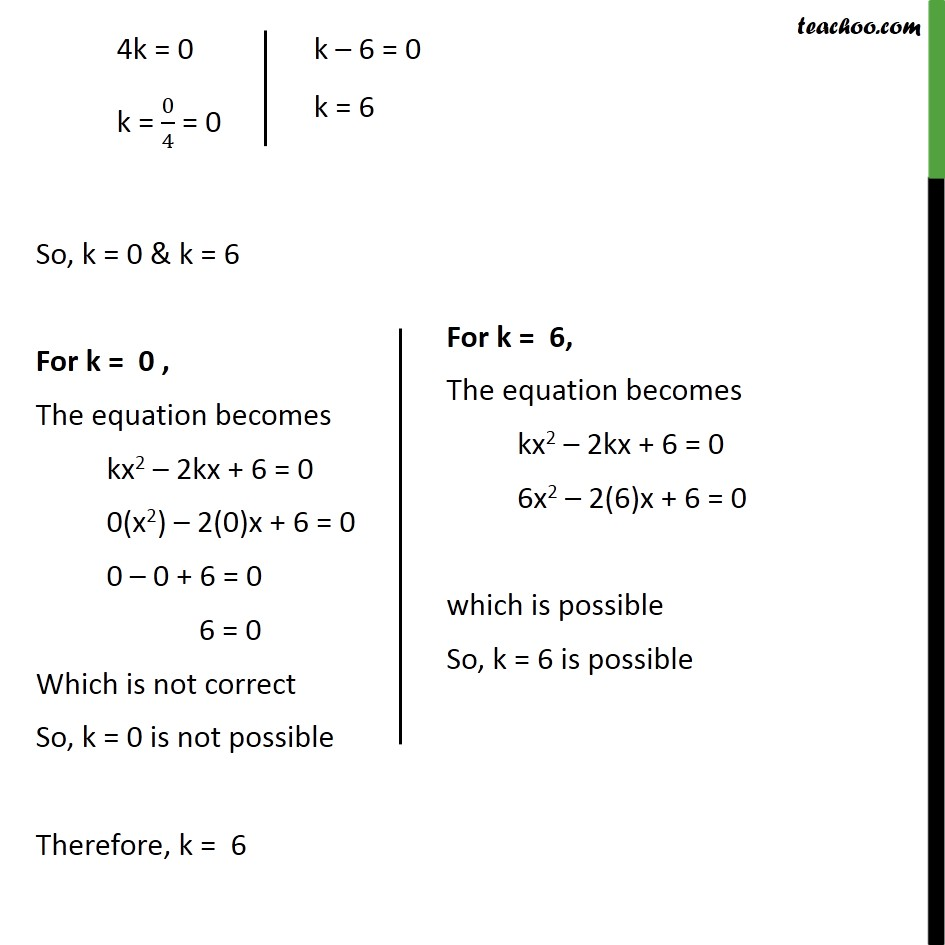Subscribe to our Youtube Channel - https://you.tube/teachoo

1. Chapter 4 Class 10 Quadratic Equations
2. Serial order wise
3. Ex 4.4

Transcript

Ex 4.4 ,2 Find the values of k for each of the following quadratic equations, so that they have two equal roots. (i) 2x2 + kx + 3 = 0 2x2 + kx + 3 = 0 Comparing equation with ax2 + bx + c = 0 a = 2, b = k, c = 3 Since the equation has 2 equal roots, D = 0 b2 – 4ac = 0 Putting values k2 – 4×2×3=0 k2 – 24 = 0 k2 = 24 k = ±√24 k = ± √(6 ×4) k = ± √6 × √4 k = ± √6 × √(2^2 ) k = ± √6 × 2 k = ± 2√6 Ex 4.4 ,2 Find the values of k for each of the following quadratic equations, so that they have two equal roots. (ii) kx (x – 2) + 6 = 0 kx (x – 2) + 6 = 0 kx2 – 2kx + 6 = 0 Comparing equation with ax2 + bx + c = 0 a = k, b = – 2k, c = 6 Since the equation has 2 equal roots D = 0 b2 – 4ac = 0 Putting values (–2k)2 – 4×𝑘×6=0 4k2 – 24k = 0 4k(k – 6) = 0 So, k = 0 & k = 6 For k = 0 , The equation becomes kx2 – 2kx + 6 = 0 0(x2) – 2(0)x + 6 = 0 0 – 0 + 6 = 0 6 = 0 Which is not correct So, k = 0 is not possible Therefore, k = 6

Ex 4.4